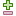## 5. The spreadsheet formula editor

### NoteAs of XMLmind XML Editor v5.8, the integrated spreadsheet engine is implemented as an add-on. Therefore, what follows is available only when add-on "Integrated spreadsheet engine" has been installed.

Formula

Type a formula in this text area.

Unlike in most spreadsheet software,

• A formula can contain spaces.

• Use of local variables and intermediate formulas is allowed.

• Comment lines are allowed and must start with '#'.

• Use newlines to separate intermediate formulas and comment lines.

Example:

```vat = `document("tutorial/VATrates.html#france_vat", .)`
# Trim the '%' sign at the end of vat.
= left(vat, len(vat) - 1)```

### Warning

Do not use names for your local variables that look like cell references. Example: '`x`' will work, but not '`x1`'. That is, [a-zA-Z][a-zA-Z]*[1-9][1-9]* (as well as true/TRUE/false/FALSE — the formula language is case insensitive) are reserved identifiers.Help about selected function

Select a function name in the Formula text area and click on this button to switch to the other tab and display online help about the selected function. Shortcut: F1.Evaluate selected text

Select an expression in the Formula text area and click on this button to evaluate it and display a dialog box containing the result of this evaluation. Shortcut: F2.

This is handy if you want to experiment with a predefined function you don't really understand.Evaluate formula

Click on this button to evaluate the whole formula and display a dialog box containing the result of this evaluation. Shortcut: F3.

Format

The format fields are useful to separate calculation from formatting.

Use case: let's suppose your formula computes an amount of money. You need to insert in the document this amount nicely formatted, preceded by string "`Total: `" and followed by string "` (excluding taxes)`".

Of course, this can be done like this:

```amount = ...
= "Total: " & numbervalue(amount, "0.00") & " (excluding taxes)"```

An alternative is to use the Format fields. First field contains a prefix which is prepended to the formatted result. Second field contains a date or number format used to format the raw result. A combobox allows to specify the locale used to interpret this format. Third field contains a suffix which is appended to the formatted result.

Therefore, specify "`Total: `" in the first field. Choose "#,##0.00" from the combobox. Specify "` (excluding taxes)`" in the third field.

Change value of attribute

A formula computes a value. This value can be used to add/replace the text node immediately after the formula or this value can be used to add/replace an attribute of the element containing the formula.

If you want to use a formula to change an attribute, check this toggle and use the associated combobox to specify the name of this attribute.

Disabled

Check this toggle to disable the formula being edited.

Disabling a formula means passivating it. That is, it is no longer used to update the document. In some cases, this may be a handy alternative to removing it.

### Tip

In the styled view, formulas are represented by a small F icon. Clicking on this icon with the middle button of the mouse allows to switch the state of the formula from enabledto disabledand vice-versa.